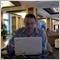# A PitchFork Price function to return price at any point and deviation from median available for general use16

UPDATE - Apologies I have now found a bug in the deviation line calculation. In the process of rectifying it now. I'll repost the function when it's fixed

UPDATE - Looks to be working correctly now, had incorrect variable as start point for the deviation line.

Hi All,

I recently had a requirement to obtain prices from a pitchfork, and more so trend lines running parallel in between the forks at x% deviations. Realising this is no straight forward task due to no GetValueByShift for pitchforks and having searched a fair bit for a function that calculates the pitchforks manually without much luck (apologies if it does exist), I thought I would set about building a function that could do it for me without the requirement for drawing trend lines and using GetValueByShift i.e pure calculation of the price. As I needed it for an EA, not having to draw the trend lines is a much better outcome in my opinion for initial back testing. Plus for me I would prefer to be able to keep all the values and calculations in my code than rely on reading from objects.

Anyway once I finished it I thought I'd publish it as it's something I've had trouble finding for a while. I haven't been coding mt4 for long and always found lots of useful code from other people to help me learn so nice to be able to contribute something back. Apologies if there are any bugs, I haven't done extensive testing with it, but so far haven't had an issues with how I've been using it.

Usage:

t_1 = Time1 of Fork (this has to be the time of the anchor point of the fork)

p_1 = Price1 of fork (again price of the anchor point)

t_2, t_3, p_2, p_3 = These are the other prices and times. It shouldn't really matter which way round you put these in. I have built it so it will find the correct point to use for the calculations (not fully tested though)

bar = the bar number back from 0 bar you want to get the price from (0=now)

deviation = percentage above / below median to use as reference line eg 1 would be the top line of the fork, -1 would be the bottom. -0.25 would be a trend line running parallel to median 25% between median and bottom of fork. -1.25 would be 25% past the bottom of the fork etc etc

drawDeviation = draws a trendline for the deviation line

drawLines = draws a vline and hline to mark a cross hair for the price

drawFork = draws a standard pitchfork object using the price/time values parsed

That's about it, happy to take any feedback or provide any assistance.

Cheers

fridayStreet

```double GetPitchPrice(int t_1, double p_1, int t_2, double p_2, int t_3, double p_3, int bar, double deviation=0, bool drawDeviation=true, bool drawLines=false, bool drawFork=true){

if(p_2 == p_3){
Print("error pitchfork prices match p1:"+p_1+" p2:"+p_2+" p3:"+p_3);
return;
}
if(t_1 == t_3 || t_1 == t_2){
Print("error 2 pitchfork times match t1:"+t_1+" t2:"+t_2+" t3:"+t_3);
return;
}

double p1, p2;
int b1, b2;

ArrayInitialize(p1,0);
ArrayInitialize(p2,0);
ArrayInitialize(b1,0);
ArrayInitialize(b2,0);

p1 = p_1;
p1 = p_2;
p1 = p_3;
b1 = iBarShift(Symbol(),0,t_1,0);
b1 = iBarShift(Symbol(),0,t_2,0);
b1 = iBarShift(Symbol(),0,t_3,0);

double highestPrice = p1;
if(p1 < p1) highestPrice = p1;
double pDist = MathAbs(p1-p1)/2;
p2 = p1;
if(pDist > 0) p2 = highestPrice-pDist;

int lowestBar = b1;
double lowestBarPrice = p1;
if(b1 < b1){
lowestBar = b1;
lowestBarPrice = p1;
}

double bDist  = MathAbs(b1-b1);
bDist = bDist/2;

bDist = b1-(lowestBar+bDist);

double m = ((p1-p2)/Point)/bDist;

double x = b1-lowestBar;
double y = m*x;

p2 = p1-(y*Point);
pDist = MathAbs(p2-lowestBarPrice)*deviation;

b1 = bar;
x = b1-b1;
y = m*x;
p1 = (p1-(y*Point))+pDist;
p1 = NormalizeDouble(p1,5);

int time = Time[b1];
if(drawLines){

if(ObjectFind("PitchPriceV"+time) == -1){
ObjectCreate("PitchPriceV"+time,OBJ_VLINE,0,time,p1);
ObjectSet("PitchPriceV"+time,OBJPROP_COLOR,Magenta);
}
ObjectSet("PitchPriceV"+time,OBJPROP_TIME1,time);

if(ObjectFind("PitchPriceH"+time) == -1){
ObjectCreate("PitchPriceH"+time,OBJ_HLINE,0,time,p1);
ObjectSet("PitchPriceH"+time,OBJPROP_COLOR,Magenta);
}
ObjectSet("PitchPriceH"+time,OBJPROP_PRICE1,p1);
}

if(drawDeviation){

if(ObjectFind("PitchDeviation"+deviation+time) == -1){
ObjectCreate("PitchDeviation"+deviation+time,OBJ_TREND,0,t_1,p_1+pDist,time,p1);
ObjectSet("PitchDeviation"+deviation+time,OBJPROP_COLOR,Yellow);
ObjectSet("PitchDeviation"+deviation+time,OBJPROP_STYLE,STYLE_DASH);
ObjectSet("PitchDeviation"+deviation+time,OBJPROP_WIDTH,0);
}
ObjectSet("PitchDeviation"+deviation+time,OBJPROP_TIME1,t_1);
ObjectSet("PitchDeviation"+deviation+time,OBJPROP_PRICE1,p_1+pDist);
ObjectSet("PitchDeviation"+deviation+time,OBJPROP_TIME2,time);
ObjectSet("PitchDeviation"+deviation+time,OBJPROP_PRICE2,p1);
}

if(drawFork){
if(ObjectFind("PitchFork"+time) == -1){
ObjectCreate("PitchFork"+time,OBJ_PITCHFORK,0,t_1,p_1,t_2,p_2,t_3,p_3);
ObjectSet("PitchFork"+time,OBJPROP_COLOR,Red);
}
ObjectSet("PitchFork"+time,OBJPROP_TIME1,t_1);
ObjectSet("PitchFork"+time,OBJPROP_PRICE1,p_1);
ObjectSet("PitchFork"+time,OBJPROP_TIME2,t_2);
ObjectSet("PitchFork"+time,OBJPROP_PRICE2,p_2);
ObjectSet("PitchFork"+time,OBJPROP_TIME3,t_3);
ObjectSet("PitchFork"+time,OBJPROP_PRICE3,p_3);
}

return(p1);
}
```17401

fridaystreet:

Hi All,

I recently had a requirement to obtain prices from a pitchfork, and more so trend lines running parallel in between the forks at x% deviations. Realising this is no straight forward task due to no GetValueByShift for pitchforks and having searched a fair bit for a function that calculates the pitchforks manually without much luck (apologies if it does exist),

Did you try GetValueByShift() with a pitchfork Object ? I always assumed it would work but have never tried it.16

No as far as I'm aware it doesn't work, besides which it wouldn't return the values of deviations of the normal lines17401

fridaystreet:
No as far as I'm aware it doesn't work, besides which it wouldn't return the values of deviations of the normal lines
OK, seems you are correct https://www.mql5.com/en/forum/1325937

Dear fridaystreet,

You have developed good function to get pitchfork median line value.

I guess your function does not return the value of other two lines of the fork.

Can you please write a function which returns other 2 lines value as well.

Thanks,

AmitModerator
123036

----------------

This thread is educational and the aim is to educate thread members in the correct way to manually take pips from the market.   It will be suited to all grade and types of trader.

·         It will suit the busy professional who only has 15 minutes before breakfast to place his/her trade before leaving for the office;

·         It will suit the busy mother/father who has to be up and down feeding the baby and cleaning the house;

·         It will suit the trader who has the commitment but has never been shown the direction;

·         It will suit those who are tired of getting their account blown by service providers;

·         it will suit those who are tired of blowing their own account.